Ex 9.5

Chapter 9 Class 12 Differential Equations
Serial order wise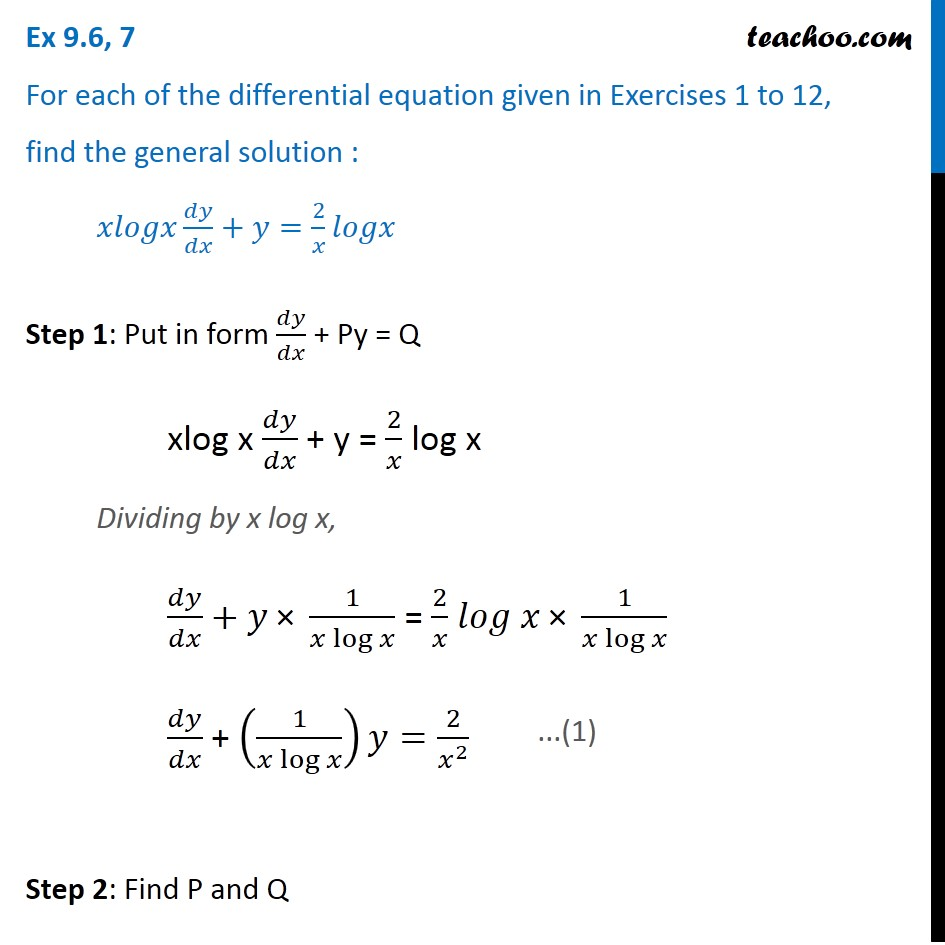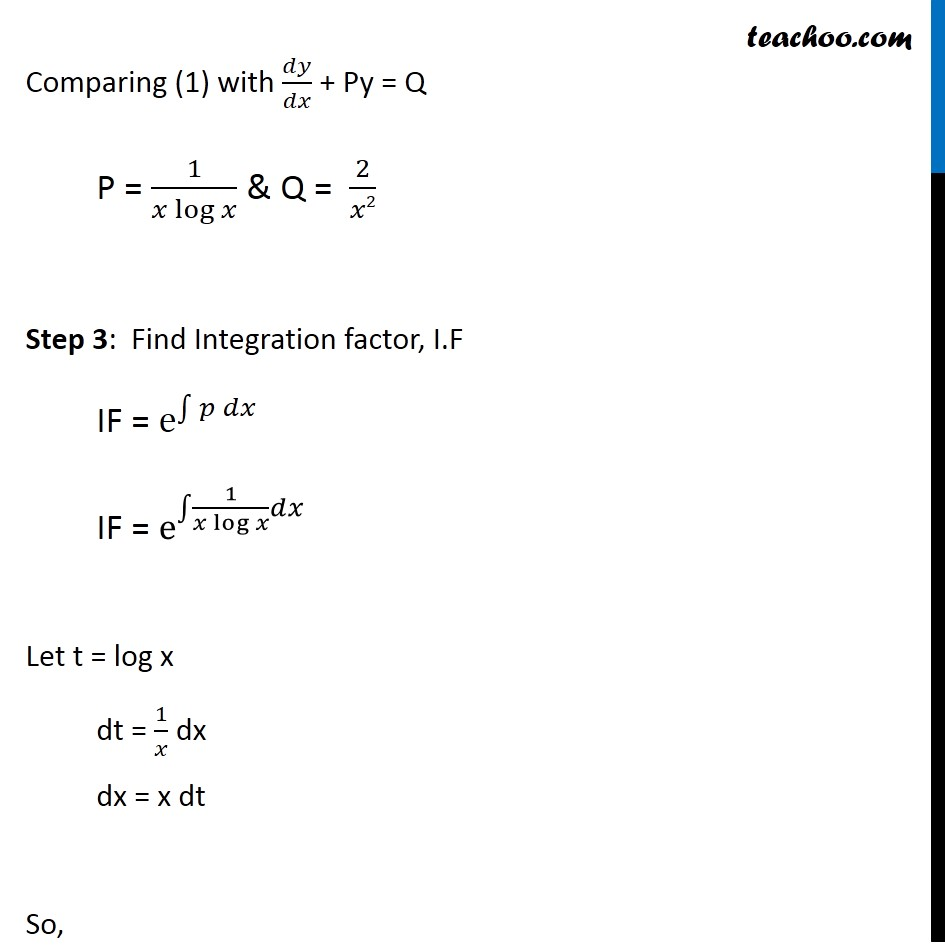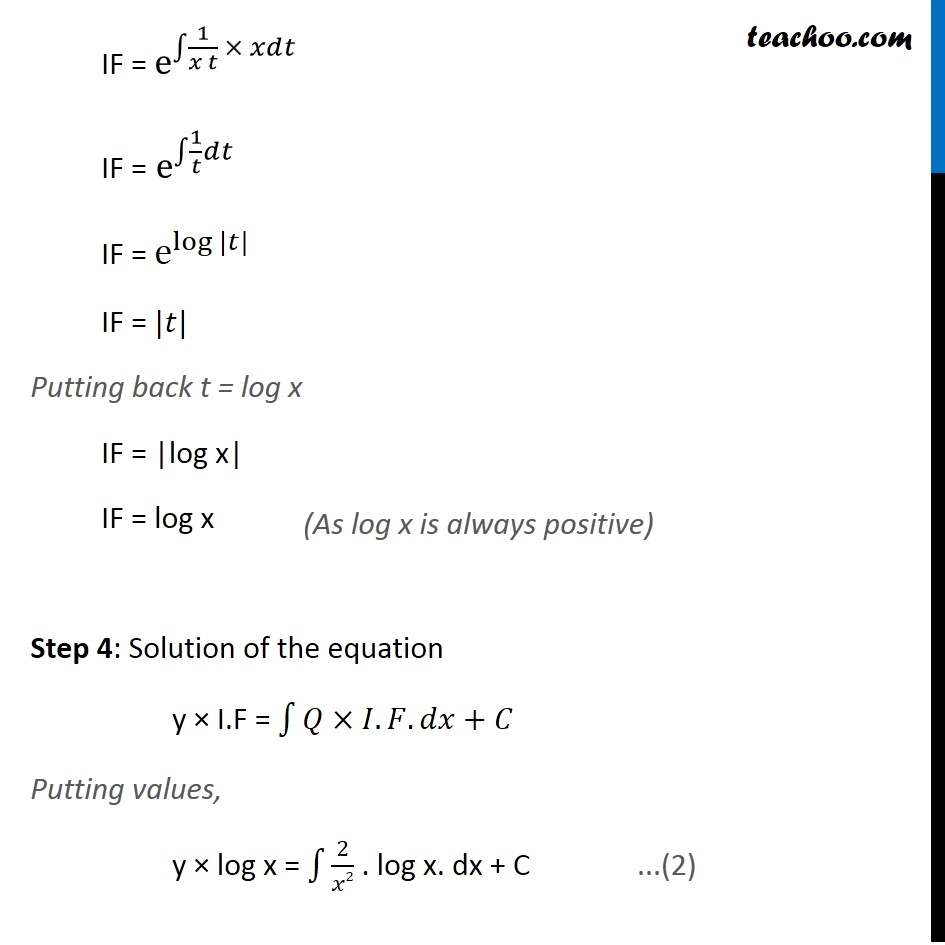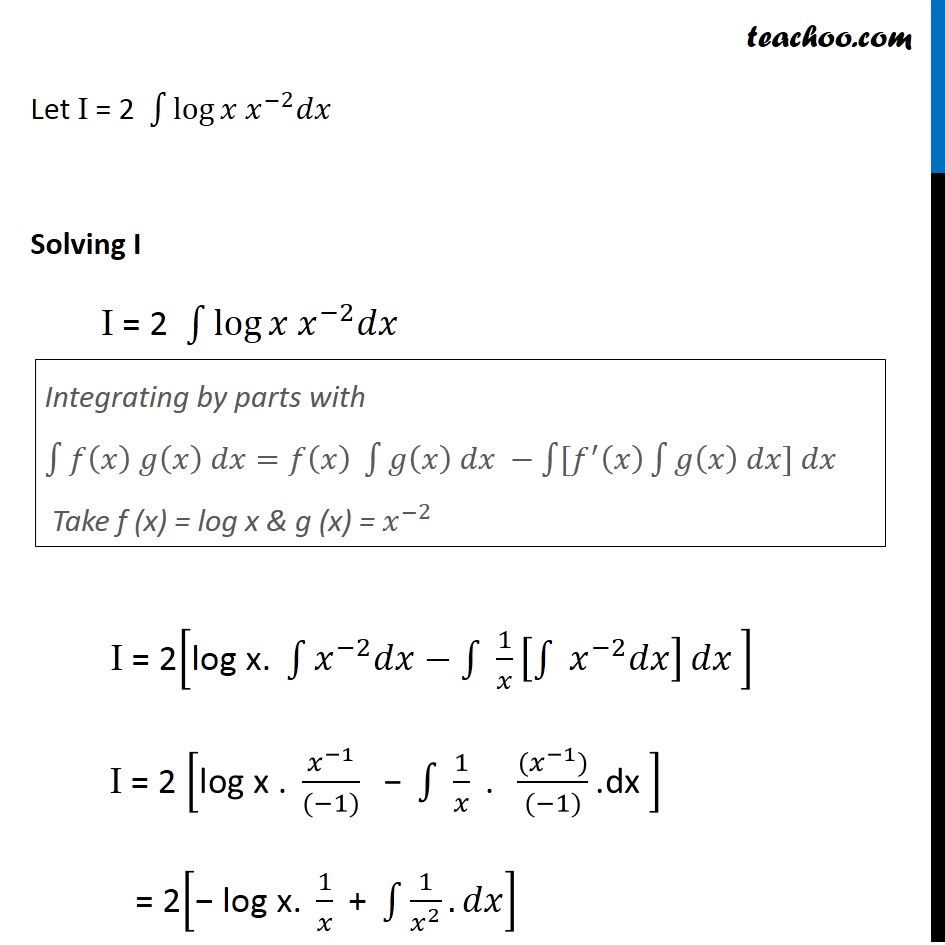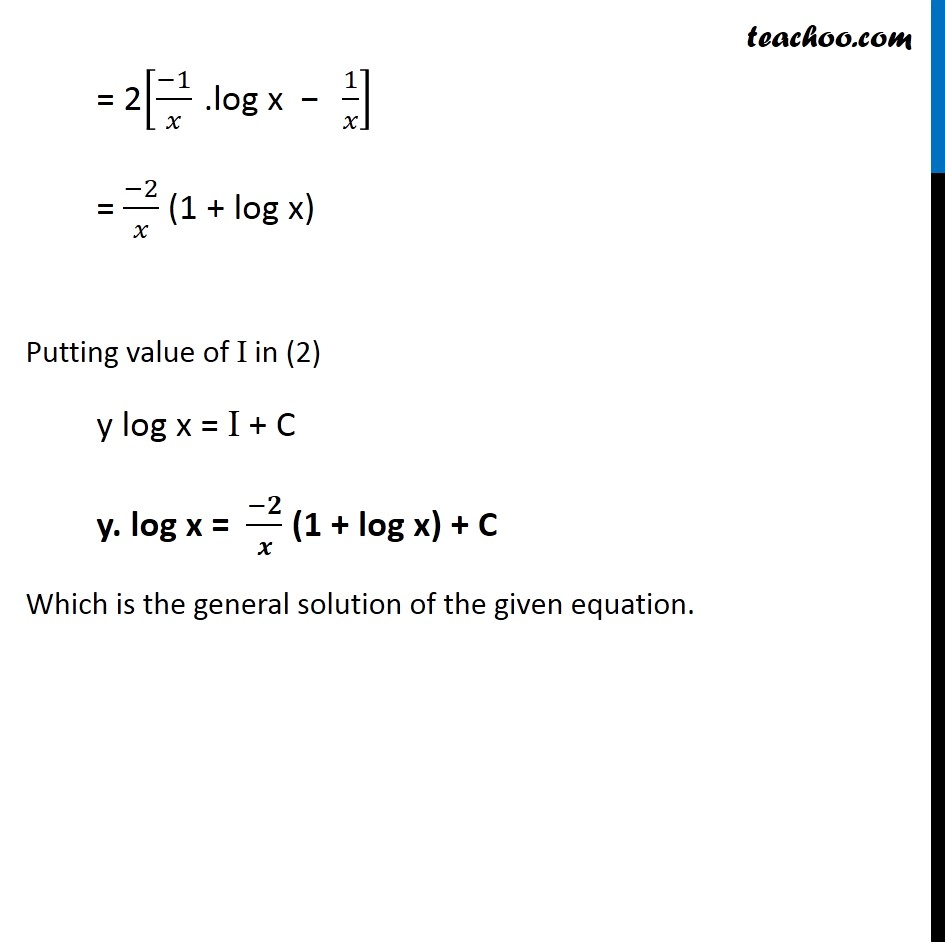Learn in your speed, with individual attention - Teachoo Maths 1-on-1 Class

### Transcript

Ex 9.5, 7 For each of the differential equation given in Exercises 1 to 12, find the general solution : 𝑥𝑙𝑜𝑔𝑥 𝑑𝑦/𝑑𝑥+𝑦=2/𝑥 𝑙𝑜𝑔𝑥 Step 1: Put in form 𝑑𝑦/𝑑𝑥 + Py = Q xlog x 𝑑𝑦/𝑑𝑥 + y = 2/𝑥 log x Dividing by x log x, 𝑑𝑦/𝑑𝑥+𝑦" × " 1/(𝑥 log⁡𝑥 ) = 2/𝑥 𝑙𝑜𝑔 𝑥" × " 1/(𝑥 log⁡𝑥 ) 𝑑𝑦/𝑑𝑥 + (1/(𝑥 log⁡𝑥 ))𝑦=2/𝑥^2 Step 2: Find P and Q ...(1) Comparing (1) with 𝑑𝑦/𝑑𝑥 + Py = Q P = 1/(𝑥 log⁡𝑥 ) & Q = 2/𝑥2 Step 3: Find Integration factor, I.F IF = e^∫1▒〖𝑝 𝑑𝑥〗 IF = e^∫1▒〖1/(𝑥 log⁡𝑥 ) 𝑑𝑥〗 Let t = log x dt = 1/𝑥 dx dx = x dt So, IF = e^∫1▒〖1/(𝑥 𝑡) × 𝑥𝑑𝑡〗 IF = e^∫1▒〖1/𝑡 𝑑𝑡〗 IF = e^log⁡〖|𝑡|〗 IF = |𝑡| Putting back t = log x IF = |log x| IF = log x Step 4: Solution of the equation y × I.F = ∫1▒〖𝑄×𝐼.𝐹. 𝑑𝑥+𝐶〗 Putting values, y × log x = ∫1▒2/𝑥2 . log x. dx + C (As log x is always positive) ...(2) Let I = 2 ∫1▒log⁡〖𝑥 𝑥^(−2) 𝑑𝑥〗 Solving I I = 2 ∫1▒log⁡〖𝑥 𝑥^(−2) 𝑑𝑥〗 I = 2["log x. " ∫1▒〖𝑥^(−2) 𝑑𝑥−∫1▒ 1/𝑥 [∫1▒〖 𝑥^(−2) 𝑑𝑥〗] 〗 𝑑𝑥" " ] I = 2 ["log x . " 𝑥^(−1)/((−1)) " − " ∫1▒〖 1/𝑥〗 " . " ((𝑥^(−1)))/((−1)) ".dx " ] = 2["− log x. " 1/𝑥 " + " ∫1▒〖1/𝑥^2 .𝑑𝑥〗] Integrating by parts with ∫1▒〖𝑓(𝑥) 𝑔(𝑥) 𝑑𝑥=𝑓(𝑥) ∫1▒〖𝑔(𝑥) 𝑑𝑥 −∫1▒〖[𝑓^′ (𝑥) ∫1▒〖𝑔(𝑥) 𝑑𝑥] 𝑑𝑥〗〗〗〗 Take f (x) = log x & g (x) = 𝑥^(−2) = 2[(−1)/𝑥 " .log x − " 1/𝑥] = (−2)/𝑥 (1 + log x) Putting value of I in (2) y log x = I + C y. log x = (−𝟐)/𝒙 (1 + log x) + C Which is the general solution of the given equation.